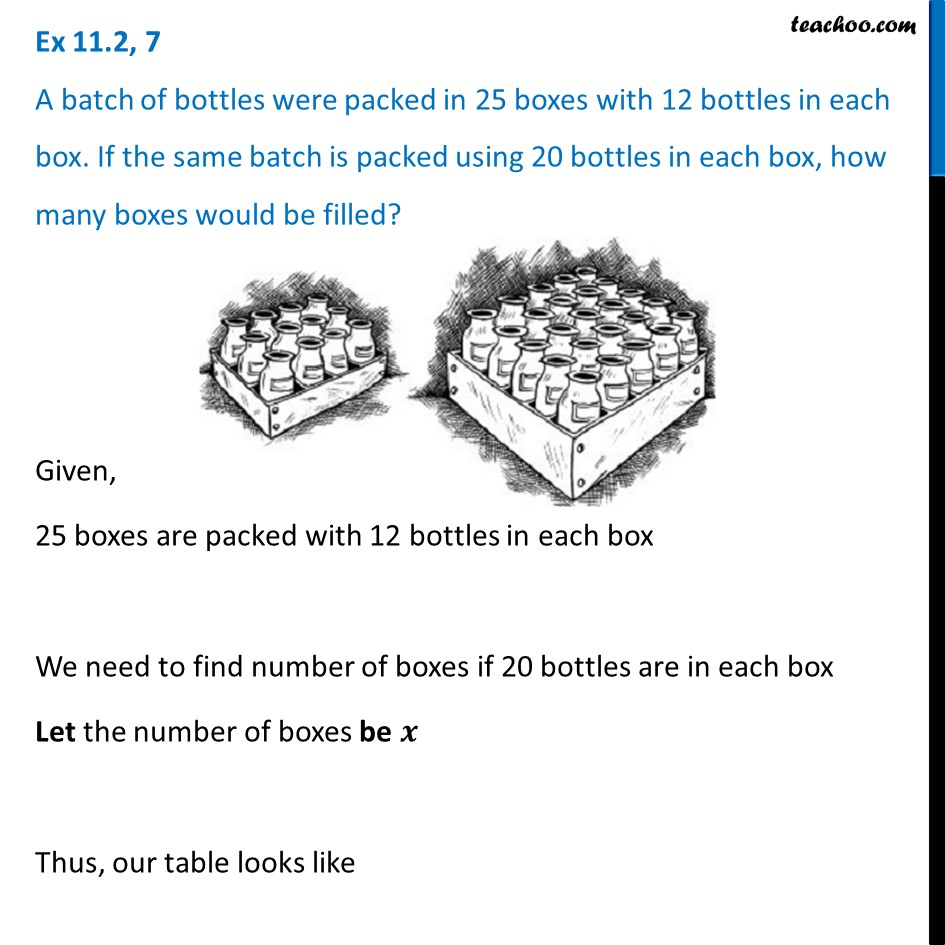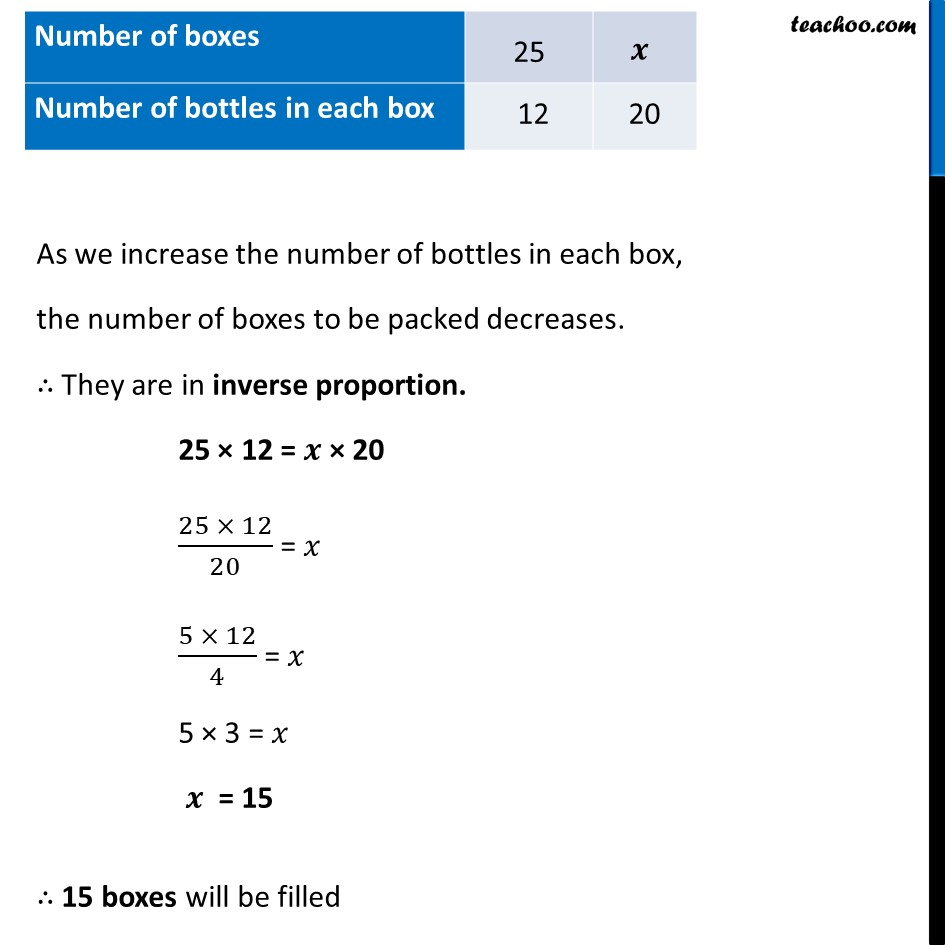Ex 11.2

Chapter 11 Class 8 Direct and Inverse Proportions
Serial order wiseLearn in your speed, with individual attention - Teachoo Maths 1-on-1 Class

### Transcript

Ex 11.2, 7 A batch of bottles were packed in 25 boxes with 12 bottles in each box. If the same batch is packed using 20 bottles in each box, how many boxes would be filled?Given, 25 boxes are packed with 12 bottles in each box We need to find number of boxes if 20 bottles are in each box Let the number of boxes be 𝒙 Thus, our table looks like As we increase the number of bottles in each box, the number of boxes to be packed decreases. ∴ They are in inverse proportion. 25 × 12 = 𝒙 × 20 (25 × 12)/20 = 𝑥 (5 × 12)/4 = 𝑥 5 × 3 = 𝑥 𝒙 = 15 ∴ 15 boxes will be filled